# Transposed Convolutions explained with… MS Excel!

You’ve successfully navigated your way around 1D Convolutions, 2D Convolutions and 3D Convolutions. You’ve conquered multi-input and multi-output channels too. But for the last blog post in the convolution series we’re onto the boss level: understanding the transposed convolution.

So let’s start with the name and see what we’re dealing with. A transpose “causes (two or more things) to change places with each other”. When we’re transposing matrices we change the order of their dimensions, so for a 2D matrix we essentially ‘flip’ values with respect to the diagonal. We won’t be covering this in the series, but it’s possible to represent operations (such as rotations, translations, and convolutions) as matrices. See Section 4.1 of Dumoulin & Visin if you’re interested. When we’re transposing convolutions we change the order of the dimensions in this convolution operation matrix, which has some interesting effects and leads to different behaviours to the regular convolutions we’ve learnt about so far.

Sometimes you’ll see this operation referred to as a ‘deconvolution’ but they are not equivalent. A deconvolution attempts to reverse the effects of a convolution. Although transposed convolutions can be used for this, they are more flexible. Other valid names for transposed convolutions you might see in the wild are ‘fractionally strided convolutions’ and ‘up convolutions’.

# Why do we need them?

One of the best ways for us to gain some intuition is by looking at examples from Computer Vision that use the transposed convolution. Most of these examples start with a series of regular convolutions to compress the input data into an abstract spatial representation, and then use transposed convolutions to decompress the abstract representation into something of use.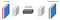Figure 1: Auto-encoding an RGB image with two Conv2D followed by two Conv2DTranspose. (source)

A convolutional auto-encoder is tasked with recreating its input image, after passing intermediate results through a ‘bottleneck’ of a limited size. Uses of auto-encoders include compression, noise removal, colourisation and in-painting. Success depends on being able to learn dataset specific compression in the convolution kernels and dataset specific decompression in the transposed convolution kernels. Why stop there though?

With ‘super resolution’ the objective is to upscale the input image to higher resolutions, so transposed convolutions can be used as an alternative to classical methods such as bicubic interpolation. Similar arguments to convolutions using learnable kernels over hand crafted kernels apply here.

Semantic segmentation is an example of using transposed convolution layers to decompress the abstract representation into a different domain (from the RGB image input). We output a class for each pixel of the input image, and then just for visualisation purposes, we render each class as a distinct colour (e.g. the person class shown in red, cars in dark blue, etc.).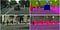Figure 3: Semantic segmentation of Cityscapes, with input on left and output on right. (source)

Clearly transposed convolutions are more flexible than classical upsampling methods (like bicubic or nearest neighbour interpolation), but there are a few disadvantages. You can’t apply transposed convolutions without learning the optimal kernel weights first, as you could with classical upsampling methods. And there can be checkerboard artifacts in the output.

Advanced: to avoid checkerboard artifacts, an alternative upsampling method that’s gaining popularity is to apply classical upsampling followed by a regular convolution (that preserves the spatial dimensions).

# A spreadsheet paints a thousand formulas

Unlike for regular convolutions, where explanations are pretty consistent and diagrams are often intuitive, the world of transposed convolutions can be a little more daunting. You’ll often read different (seemingly disconnected) ways to think about the computation. So in this blog post I’ll take two mental models of transposed convolutions and help you join the dots using our trusty friend… MS Excel. And we’ll code things up in Apache MXNet because we’ll probably want to use them in practice some day!

Advanced: the transposed convolution operation is equivalent to the gradient calculation for a regular convolution (i.e. the backward pass of a regular convolution). And vice versa. Consider this while reading the next section.

# Mental Model #1: Distributing Values

Our first mental model is more intuitive (at least for me), and we’ll work step-by-step towards the second mental model that’s closer to how transposed convolutions are implemented in deep learning frameworks.

Let’s start from the perspective of a single value in the input. We take this value and ‘distribute’ it to a neighbourhood of points in the output. A kernel defines exactly how we do this, and for each output cell we multiply the input value by the corresponding weight of the kernel. We repeat this process for every value in the input, and accumulate values in each output cell. Check out Figure 4 for an example of this accumulation (with unit input and kernel).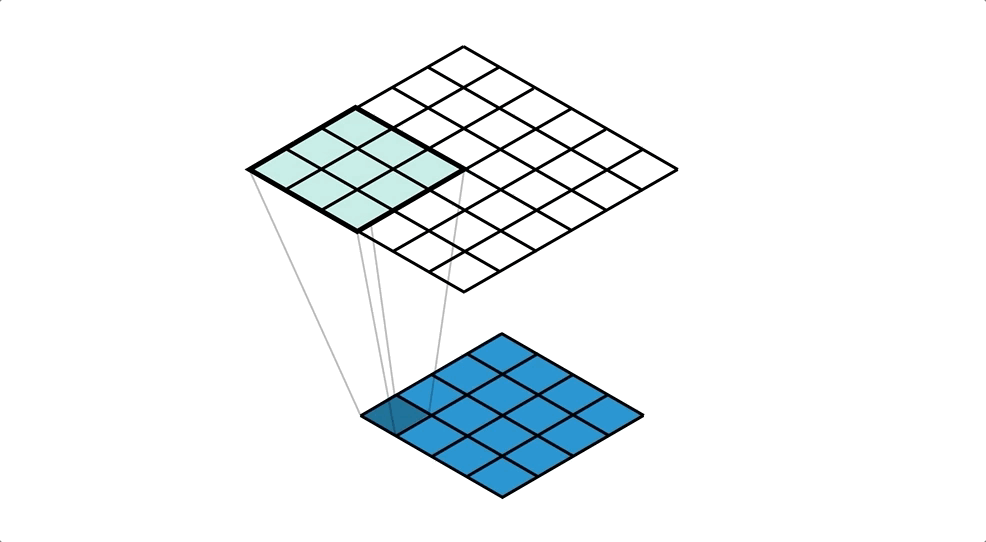Figure 4: A Conv2DTranspose with 3x3 kernel (not seen explicitly) applied to a 4x4 input to give a 6x6 output.

Our kernel values are hidden in the animation above, but it is important to understand that the kernel is defining the amount of the input value that’s being distributed to each of the output cells (in the neighbourhood). We can see this more clearly in the spreadsheet in Figure 5, even with a unit kernel.

Advanced: if you’re observant you may have spotted that the edges of the output get less accumulation than the centre cells. Often this isn’t an issue because kernel weights learn to adjust for this and can be negative too.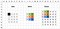Figure 5: A Conv2DTranspose with 3x3 kernel (seen explicitly) applied to a 4x4 input to give a 6x6 output.

With Apache MXNet we can replicate this using the `Transpose` blocks. We have got two spatial dimensions so we’ll use `Conv2DTranspose`. Similarly MXNet defines `Conv1DTranspose` and `Conv3DTranspose`.

`input_data = mx.nd.ones(shape=(4,4))kernel = mx.nd.ones(shape=(3,3))conv = mx.gluon.nn.Conv2DTranspose(channels=1, kernel_size=(3,3))# see appendix for definition of `apply_conv`output_data = apply_conv(input_data, kernel, conv)print(output_data)# [[[[1. 2. 3. 3. 2. 1.]#    [2. 4. 6. 6. 4. 2.]#    [3. 6. 9. 9. 6. 3.]#    [3. 6. 9. 9. 6. 3.]#    [2. 4. 6. 6. 4. 2.]#    [1. 2. 3. 3. 2. 1.]]]]# <NDArray 1x1x6x6 @cpu(0)>`

# Mental Model #2: Collecting Values

Another way of thinking about transposed convolutions is from the perspective of a cell in the output, rather than a value in the input as we did with first mental model. When we do this we end up with something strangely familiar, something very similar to a regular convolution!

One step at a time we’ll convert what we already know to this new way of thinking. We start with the animation in Figure 6. It highlights a single cell in the output, and looks at the input values that distribute into it. You should pay close attention to the kernel weights used for each of the input values.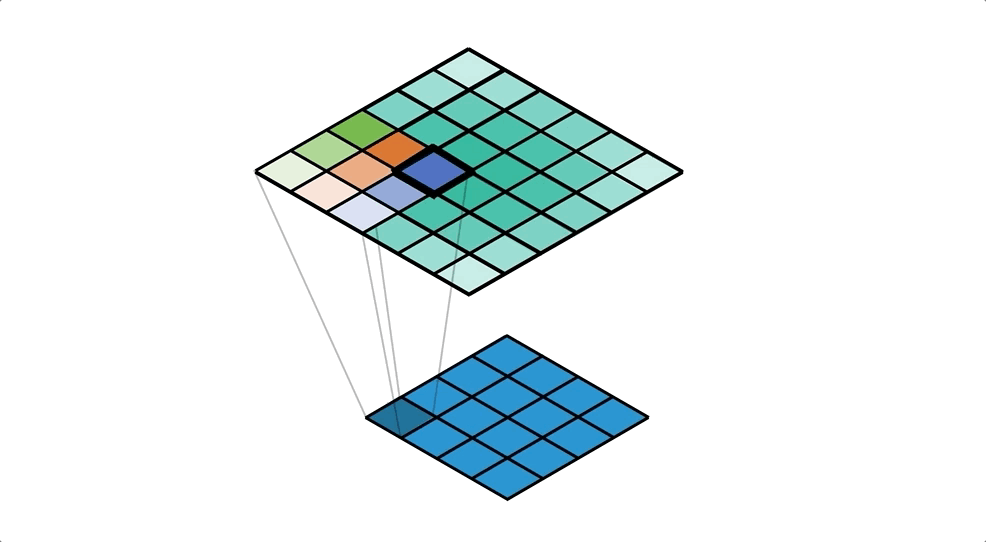Figure 6: Calculating the value of a single output cell through accumulation. Showing distribution kernel.

We can make this even more obvious in Figure 7 by colour coding the input values by the kernel weight that they get multiplied with before the accumulation. You should notice how the kernel on the input has ‘flipped’ about the centre; i.e. the dark blue weight of the kernel was bottom right when distributing, but it’s moved to top left when we think about collecting.

We’ve just created a convolution! Check the freeze frame in Figure 8 if you don’t believe me. We’re using the ‘flipped’ kernel, that despite the name, ‘transposed convolution’, isn’t actually a transpose of the distribution kernel.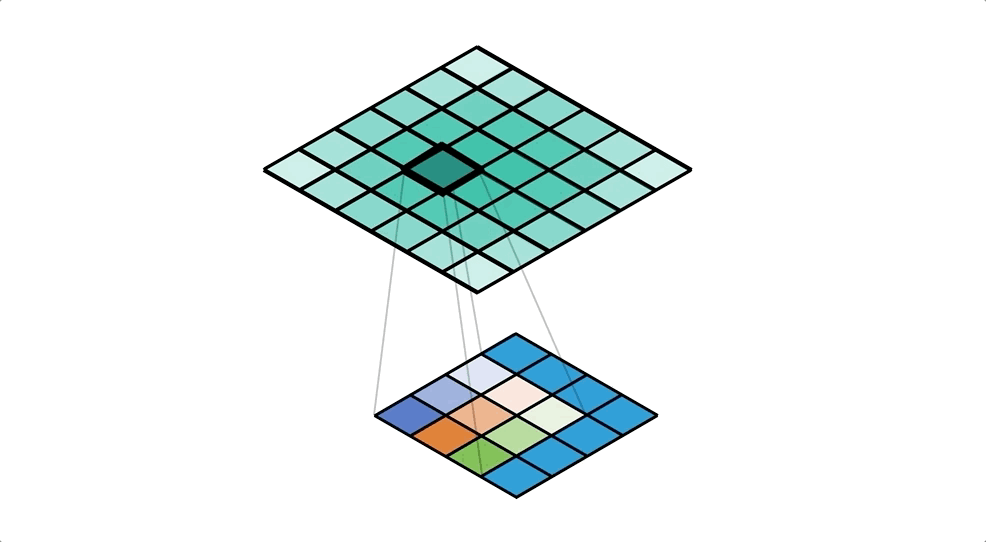Figure 8: A Conv2D equivalent to distribution seen in Figure 6.

Advanced: if you’re observant you may have spotted that applying the `Conv2D` like so would actually result in a 2x2 output. `Conv2DTranspose` with no padding is equivalent to having 2x2 padding (`(kernel_size + 1) / 2`) now that we have mapped the operation to a `Conv2D`, giving us a 6x6 output as we had before.

Collecting values with 2D Convolutions allows us to write explicit formulas for the output: ideal for MS Excel and also code implementations too. So we’d have the following formula for the top left cell of the output: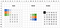Figure 10: A Conv2DTranspose with 3x3 kernel applied to a 4x4 input to give a 6x6 output. Surprisingly the padding for Conv2DTranspose is set to 0 in this example, even though implicit padding is seen.

We can confirm our results with the Apache MXNet code seen previously:

`# define input_data and kernel as above# input_data.shape is (4, 4)# kernel.shape is (3, 3)conv = mx.gluon.nn.Conv2DTranspose(channels=1, kernel_size=(3,3))output_data = apply_conv(input_data, kernel, conv)print(output_data)# [[[[ 1.  5. 11. 14.  8.  3.]#    [ 1.  6. 15. 18. 12.  3.]#    [ 4. 13. 21. 21. 15. 11.]#    [ 5. 17. 28. 27. 25. 11.]#    [ 4.  7.  9. 12.  8.  6.]#    [ 6.  7. 14. 13.  9.  6.]]]]# <NDArray 1x1x6x6 @cpu(0)>`

# GNIDDAP!

We’ve just seen a strange example of a `Conv2DTranspose` with no padding (appearing to have padding of 2x2 when thinking about it as a `Conv2D`) but things get even more mysterious when we start adding padding.

With regular convolutions, padding is applied to the input which has the effect of increasing the size of the output. With transposed convolutions, padding has the reverse effect and it decreases the size of the output. So I’m coining ‘gniddap’ in the hope you’ll remember the reverse ‘padding’.

`for pad in range(3):    conv = mx.gluon.nn.Conv2DTranspose(channels=1,                                       kernel_size=(3,3),                                       padding=(pad,pad))    output_data = apply_conv(input_data, kernel, conv)    print("With padding=({pad}, {pad}) the output shape is {shape}"          .format(pad=pad, shape=output_data.shape))# With padding=(0, 0) the output shape is (1, 1, 6, 6)# With padding=(1, 1) the output shape is (1, 1, 4, 4)# With padding=(2, 2) the output shape is (1, 1, 2, 2)`

We can think about padding for transposed convolutions as the amount of padding that’s included in the complete output. Sticking with our usual example (where the complete output is 6x6), when we define padding of 2x2 we’re essentially saying that we don’t care about the outer cells of the output (with width of 2) because that was just padding, leaving us with a 2x2 output. When thinking about transposed convolutions as regular convolutions we remove padding from the input by the defined amount. See Figure 11 for an example with MS Excel, and notice how the outputs are identical to the central values of the output in Figure 10 when there was no padding.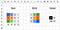Figure 11: A Conv2DTranspose with 3x3 kernel and padding of 2x2 applied to a 4x4 input to give a 6x6 output.
`# define input_data and kernel as above# input_data.shape is (4, 4)# kernel.shape is (3, 3)conv = mx.gluon.nn.Conv2DTranspose(channels=1,                                   kernel_size=(3,3),                                   padding=(2,2))output_data = apply_conv(input_data, kernel, conv)print(output_data)# [[[[21. 21.]#    [28. 27.]]]]# <NDArray 1x1x2x2 @cpu(0)>`

# SEDIRTS!

Strides are also reversed. With regular convolution we stride over the input, resulting in a smaller output. But when thinking about transposed convolutions from a distribution perspective, we stride over the output, which increases the size of the output. Strides are responsible for the upscaling effect of transposed convolutions. See Figure 12.

Advanced: checkerboard artifacts can be seen in the example below, which can start to become an issue when using strides (even after stacking multiple layers).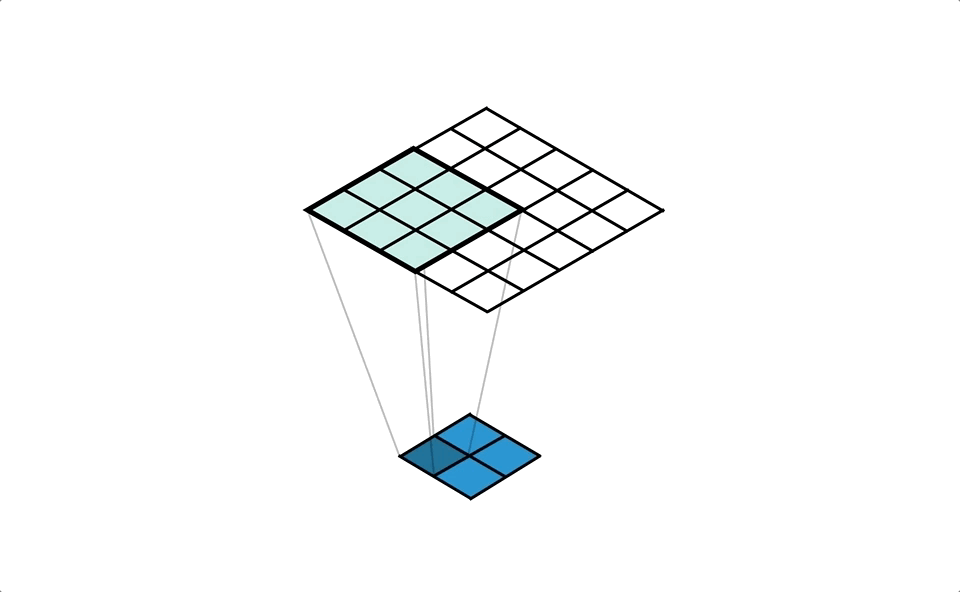Figure 12: A Conv2DTranspose with 3x3 kernel and stride of 2x2 applied to a 2x2 input to give a 5x5 output.

Although things are clear from the distributional perspective above, things get a little strange when we think about things from a collection perspective and try to implement this using a convolution. Stride over the output is equivalent to a ‘fractional stride’ over the input, and this is where the alternative name for transposed convolutions called ‘fractionally strided convolutions’ comes from. A stride of 2 over the output would be equivalent to a stride of 1/2 over the input: a fractional stride. We implement this by introducing empty spaces between our input values, the amount proportional to the stride, and then stride by one. As a result we’re applying the kernel to a region of the input that’s smaller than the kernel itself! See Figure 13 for an example.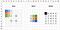Figure 13: A Conv2DTranspose with 3x3 kernel and stride of 2x2 applied to a 2x2 input to give a 5x5 output.
`# define input_data and kernel as above# input_data.shape is (2, 2)# kernel.shape is (3, 3)conv = mx.gluon.nn.Conv2DTranspose(channels=1,                                   kernel_size=(3,3),                                   strides=(2,2))output_data = apply_conv(input_data, kernel, conv)print(output_data)# [[[[ 3.  6. 12.  6.  9.]#    [ 0.  3.  0.  3.  0.]#    [ 7.  5. 16.  5.  9.]#    [ 0.  1.  0.  1.  0.]#    [ 2.  1.  4.  1.  2.]]]]# <NDArray 1x1x5x5 @cpu(0)>`

# Multi-Channel Transposed Convolutions

As with regular convolutions, each input channel will use a separate kernel and the results for each channel will be summed together to give a single output channel. We repeat this process for every output channel required, using a different set of kernels. All these kernels are kept in a single kernel array with shape:

(input channels, output channels, kernel height, kernel width)

Which is different from the kernel array shape used for a regular convolution:

(output channels, input channels, kernel height, kernel width)

`# define input_data and kernel as above# input_data.shape is (3, 5, 5)# kernel.shape is (3, 3, 3)kernel = kernel.expand_dims(0).transpose((1,0,2,3))# kernel.shape is now (3, 1, 3, 3)conv = mx.gluon.nn.Conv2DTranspose(channels=1, kernel_size=(3,3))output_data = apply_conv(input_data, kernel, conv)print(output_data)# [[[[ 4.  2.  1.  5.  2.  2.  0.]#    [ 9.  6.  7. 13.  9.  1.  4.]#    [11. 14. 12. 14. 17. 11.  4.]#    [ 5. 17. 19. 25. 18. 14.  6.]#    [ 6. 13. 25. 21. 22.  6.  6.]#    [ 1.  3. 20.  9. 17. 15.  0.]#    [ 0.  3.  4. 11. 11.  5.  2.]]]]# <NDArray 1x1x7x7 @cpu(0)>`

# Get experimental

All the examples shown in this blog posts can be found in this Excel Spreadsheet (and Google Sheet too). Click on the cells of the output to inspect the formulas and try different kernel values to change the outputs. After replicating your results in MXNet Gluon, I think you can officially add ‘convolution wizard’ as a title on your LinkedIn profile!

# Congratulations!

You’ve made it to the end of this excellent series on convolutions. I hope you learnt something useful, and now feel ready to apply these techniques to real world problems with Apache MXNet. Any questions? Just drop a comment below or check out the MXNet Discussion forum. Shares and claps would also be greatly appreciated. Many thanks!

# Appendix

## Apache MXNet

Apache MXNet (incubating) is a deep learning framework…

## Apache MXNet

Apache MXNet (incubating) is a deep learning framework designed for both efficiency and flexibility. It allows you to mix symbolic and imperative programming to maximize efficiency and productivity.

Written by

## Thom Lane

Machine Learning Scientist for AWS AI## Apache MXNet

Apache MXNet (incubating) is a deep learning framework designed for both efficiency and flexibility. It allows you to mix symbolic and imperative programming to maximize efficiency and productivity.

## More From Medium

Medium is an open platform where 170 million readers come to find insightful and dynamic thinking. Here, expert and undiscovered voices alike dive into the heart of any topic and bring new ideas to the surface. Learn more

Follow the writers, publications, and topics that matter to you, and you’ll see them on your homepage and in your inbox. Explore

If you have a story to tell, knowledge to share, or a perspective to offer — welcome home. It’s easy and free to post your thinking on any topic. Write on Medium